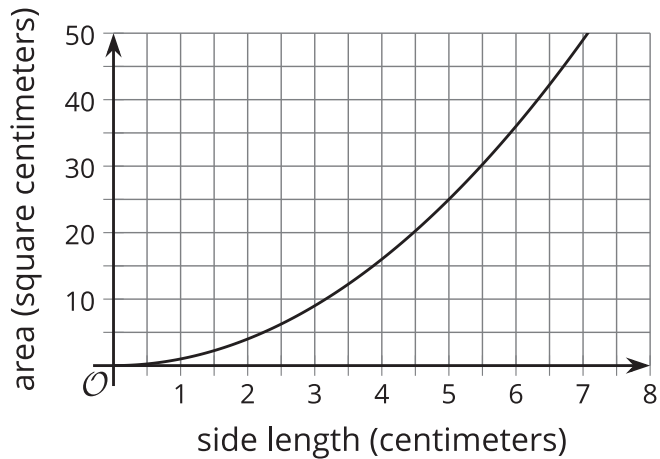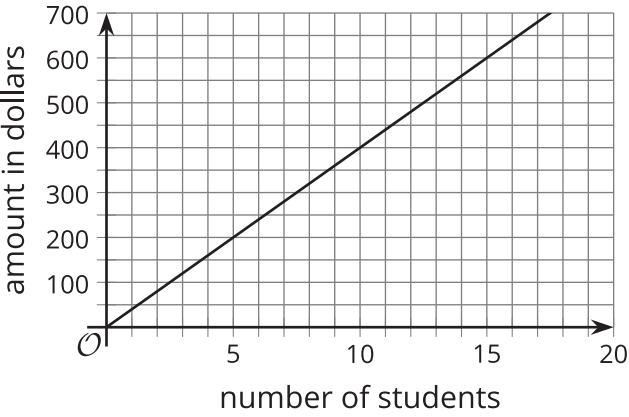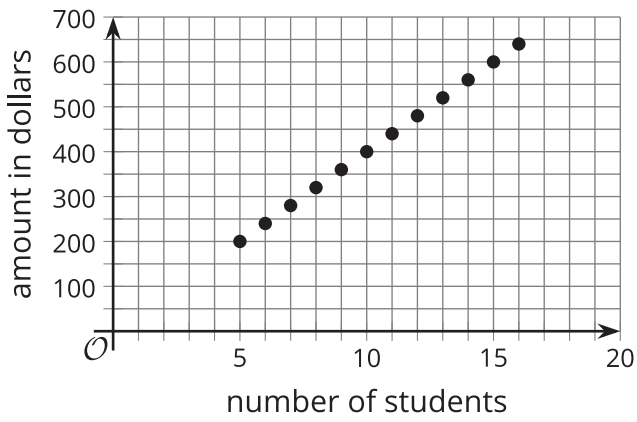# A.4.10.3 What about the Outputs?

In an earlier activity, you saw a function representing the area of a square (function A) and another representing the revenue of a tennis camp (function R). Refer to the descriptions of those functions to answer these questions. 1. Here is a graph that represents function A, defined by A(s)=s^2, where s is the side length of the square in centimeters.a. Name three possible input-output pairs of this function. b. Earlier we describe the set of all possible input values of A as “any number greater than or equal to 0.” How would you describe the set of all possible output values of A?

2. Function R is defined by R(n)=40n, where n is the number of campers. a. Is 20 a possible output value in this situation? What about 100? Explain your reasoning. b. Here are two graphs that relate number of students and camp revenue in dollars. Which graph could represent function R? Explain why the other one could not represent the function.c. Describe the set of all possible output values of R.